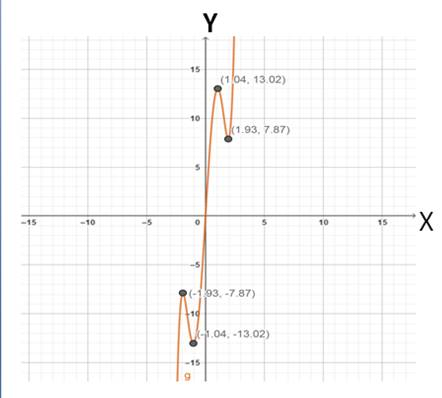# To find the local maximum and local minimum of the function### Precalculus: Mathematics for Calcu...

6th Edition
Stewart + 5 others
Publisher: Cengage Learning
ISBN: 9780840068071### Precalculus: Mathematics for Calcu...

6th Edition
Stewart + 5 others
Publisher: Cengage Learning
ISBN: 9780840068071

#### Solutions

Chapter 2.3, Problem 38E

a.

To determine

## To find the local maximum and local minimum of the function

Expert Solution

### Explanation of Solution

Given information : the function is g(x)=x58x3+20x .

Graph : Sketch the graph using graphing utility.

Step 1: Press WINDOW button to access the Window editor.

Step 2: Press Y= button.

Step 3: Enter the expression x42x311x2 which is required to graph.

Step 4: Press GRAPH button to graph the function.

Step 5: Using TRACE feature on the calculator

The graph is obtained as:From the above graph, it can be observed that the local maximum 7.86 when x1.92 , local maximum 13.02 when x1.01 and the local minimum 13.02 when x1.036 , local minimum 7.86 when x1.92

b.

To determine

### To find the interval on which the function is increasing and on which the function is decreasing up to two decimal

Expert Solution

The function f is decreasing on (1.92,1.036)(1.01,1.92) and increasing on (,1.92][1.036,1.01][1.92,) .

### Explanation of Solution

Given information :

The function is g(x)=x58x3+20x .

The graph of the function is obtained as:Using TRACE feature on the calculator,

From the above graph, it can be observed that the function f is decreasing on (1.92,1.036)(1.01,1.92) and increasing on (,1.92][1.036,1.01][1.92,)

### Have a homework question?

Subscribe to bartleby learn! Ask subject matter experts 30 homework questions each month. Plus, you’ll have access to millions of step-by-step textbook answers!